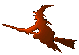Hex  1.0 Hydrogen-electron collision solver
special Namespace Reference

constant

integral

## Functions

int coulomb_zeros (double eta, int L, int nzeros, double *zeros, double epsrel=1e-8)
Get zeros of the Coulomb wave function $$F_L(-1/k,kr)$$. More...

std::vector< std::vector< int > > FdB_partition (int n)
Faa di Bruno partitioning. More...

template<class T , class OuterFunctionDerivative , class InnerFunctionDerivative >
chain_rule (OuterFunctionDerivative Df, InnerFunctionDerivative Dg, int n, double x)
Chain rule for n-th derivative. More...

## Function Documentation

template<class T , class OuterFunctionDerivative , class InnerFunctionDerivative >
 T special::chain_rule ( OuterFunctionDerivative Df, InnerFunctionDerivative Dg, int n, double x )

This function implements the Faa di Bruno's formula for n-th derivative of a nested function,

$\frac{\mathrm{d}^n}{\mathrm{d}^n x} f(g(x)) = \sum \frac{n!}{m_1! 1!^{m_1} m_2! 2!^{m_2} \dots m_n! n!^{m_n}} f^{(m_1+m_2+\dots+m_n)}(g(x)) \prod_{j=1}^n \left(g^{(j)}(x)\right)^{m_j} \ .$

The sum runs over all n-tuples $$(m_1, \dots, m_n)$$ that satisfy the Faa di Bruno's sum condition

$1 m_1 + 2 m_2 + \dots + n m_n = n \ .$

Those n-tuples are retrieved from the function FdB_partition.

 int special::coulomb_zeros ( double eta, int L, int nzeros, double * zeros, double epsrel = 1e-8 )

Calculates given number of leading zeros of the Coulomb wave function $$F_L(\eta,\rho)$$, where $$\eta = -1/k$$ and $$\rho = kr$$.

The method used comes from Ikebe Y.: The zeros of regular Coulomb wave functions and of their derivatives, Math. Comp. 29, 131 (1975) 878-887. It uses eigenvalues of a special tridiagonal matrix. The eigenvalues are computed using the standard Lapack function DSTEV .

 std::vector > special::FdB_partition ( int n )

The Faa di Bruno partitioning is computed by looping over possible n-tuples

$(m_1, m_2, \dots, m_n)$

of integers and by picking only such that satisfy the Faa di Bruno's sum condition

$1 m_1 + 2 m_2 + \dots + n m_n = n \ .$

The initial trial partitioning is a zero tuple

$(0, 0, \dots, 0)$

and the further tuples are constructed by incrementing a corresponding multidigit number, that has a number system varying with the position. The number system base for the left-most (least significant) position is $$n + 1$$, for the next position it is $$\lceil (n + 1)/2 \rceil$$, for the next it is $$\lceil (n + 1)/3 \rceil$$, etc. For example, if $$n = 4$$, the increments are

$(0, 0, 0, 0), (1, 0, 0, 0), (2, 0, 0, 0), (3, 0, 0, 0), \mathbf{(4, 0, 0, 0)},$

$(0, 1, 0, 0), (1, 1, 0, 0), (2, 1, 0, 0), \mathbf{(3, 1, 0, 0)}, (4, 1, 0, 0),$

$(0, 2, 0, 0), (1, 2, 0, 0), \mathbf{(2, 2, 0, 0)}, (3, 2, 0, 0), (4, 2, 0, 0),$

$(0, 0, 1, 0), \mathbf{(1, 0, 1, 0)}, (2, 0, 1, 0), (3, 0, 1, 0), (4, 0, 1, 0),$

$(0, 1, 1, 0), (1, 1, 1, 0), (2, 1, 1, 0), (3, 1, 1, 0), (4, 1, 1, 0),$

$(0, 2, 1, 0), (1, 2, 1, 0), (2, 2, 1, 0), (3, 2, 1, 0), (4, 2, 1, 0),$

$\mathbf{(0, 0, 0, 1)}, \ \mathrm{etc.}$

Here, the number system are 5, 3, 2 and 2. Only those tuples in bold satisfy the sum condition and will be returned.

This function is needed by the generalized chain_rule.

Todo:
Cache results.